Question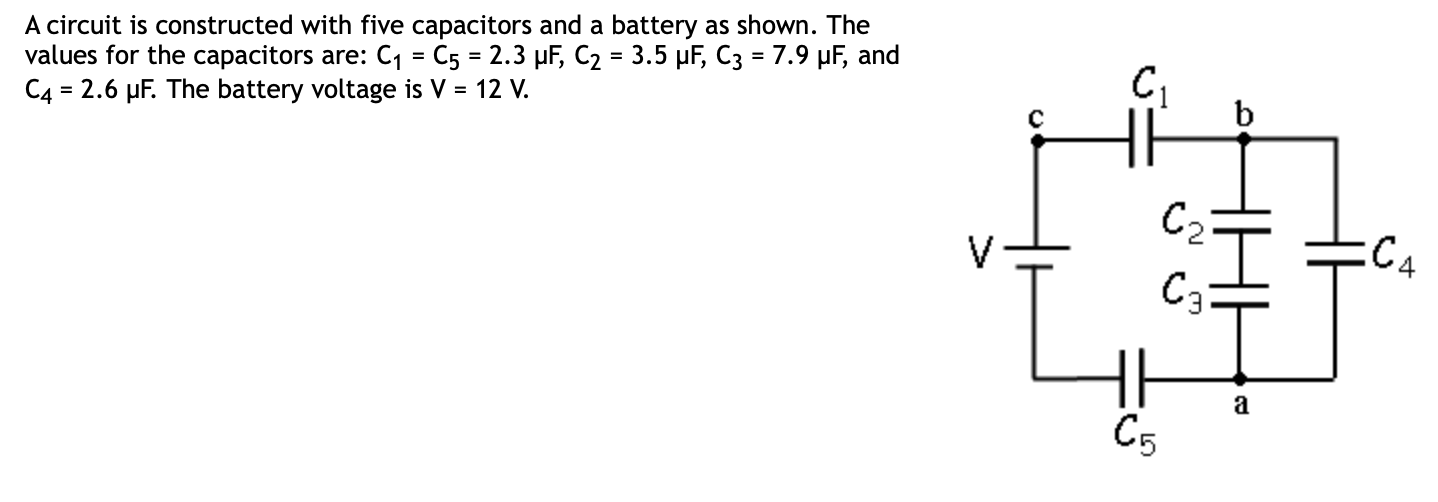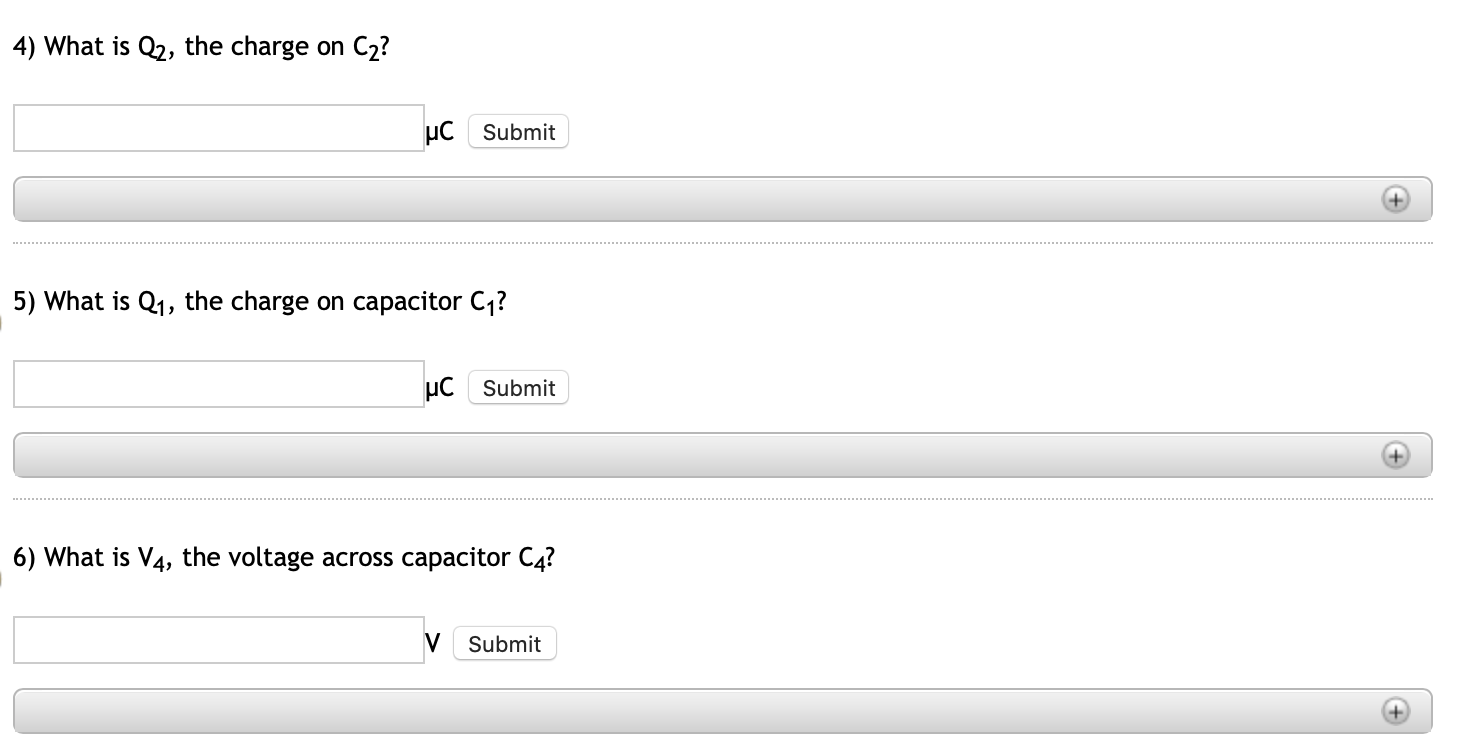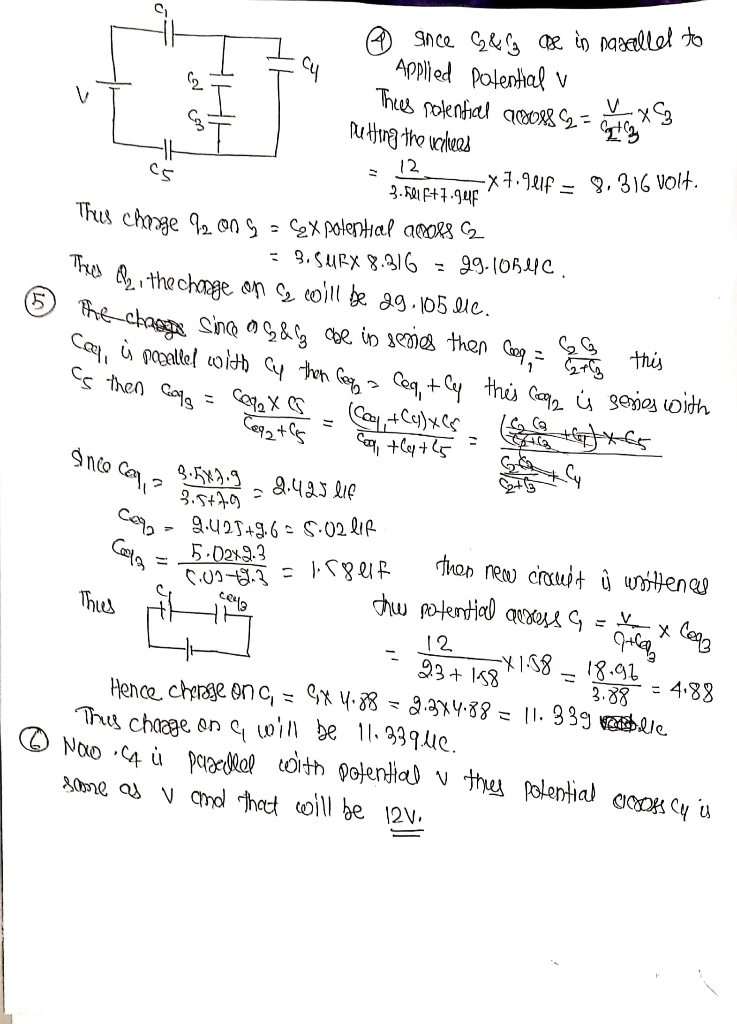#### Earn Coins

Coins can be redeemed for fabulous gifts.

Similar Homework Help Questions
• ### A circuit is constructed with five capacitors and a battery as shown. The values for...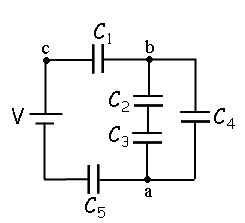A circuit is constructed with five capacitors and a battery as shown. The values for the capacitors are: C1 = C5 = 3.9 μF, C2 = 1.9 μF, C3 = 7.6 μF, and C4 = 2.2 μF. The battery voltage is V = 12 V. 4)What is Q2, the charge on C2? 5)What is Q1, the charge on capacitor C1? C1 b HH Cz C4 Ca HH a C5

• ### A circuit is constructed with five capacitors and a battery as shown. The values for the...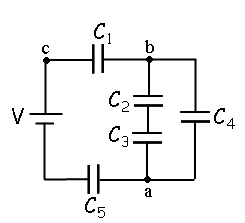A circuit is constructed with five capacitors and a battery as shown. The values for the capacitors are: C1 = C5 = 4.2 μF, C2 = 1 μF, C3 = 5.8 μF, and C4 = 4.4 μF. The battery voltage is V = 12 V. 1) What is Cab, the equivalent capacitance between points a and b?μF 2) What is Cac, the equivalent capacitance between points a and c? 3) What is Q5, the charge on capacitor C5?μC 4) What...

• ### A circuit is constructed with five capacitors and a battery as shown. The values for the...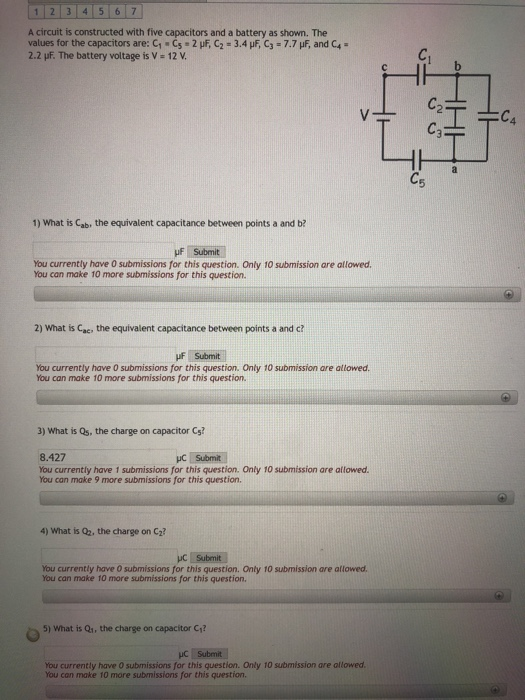A circuit is constructed with five capacitors and a battery as shown. The values for the capacitors are: C1 . С5 . 2 ,F, c,. 3.4 F, C3-77 F, and C+" 2.2 pF. The battery voltage is V 12v 1) What is Cab, the equivalent capacitance between points a and b? uF Submit You currently have O submissions for this question. Only 10 submission are allowed. You can make 10 more submissions for this question. 2) What is ac, the...

• ### Vbat = C4 C5 Consider the circuit shown above. The battery provides a voltage of Vbat...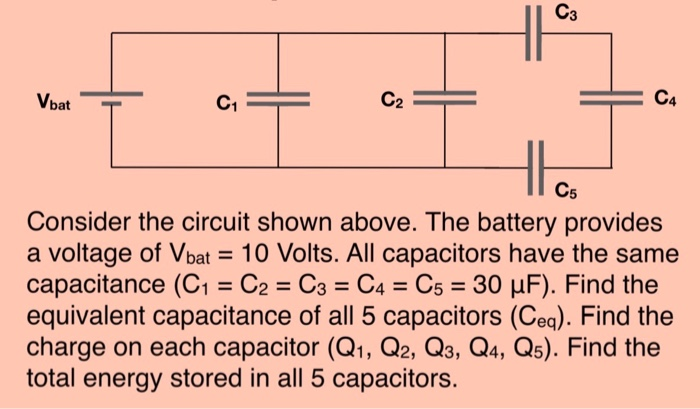Vbat = C4 C5 Consider the circuit shown above. The battery provides a voltage of Vbat = 10 Volts. All capacitors have the same capacitance (C1 = C2 = C3 = C4 = C5 = 30 uF). Find the equivalent capacitance of all 5 capacitors (Ceg). Find the charge on each capacitor (Q1, Q2, Q3, Q4, Q5). Find the total energy stored in all 5 capacitors.

• ### Problem 6. In the following capacitor circuit, the capacitors have values C1 = 1.0 uF, C2...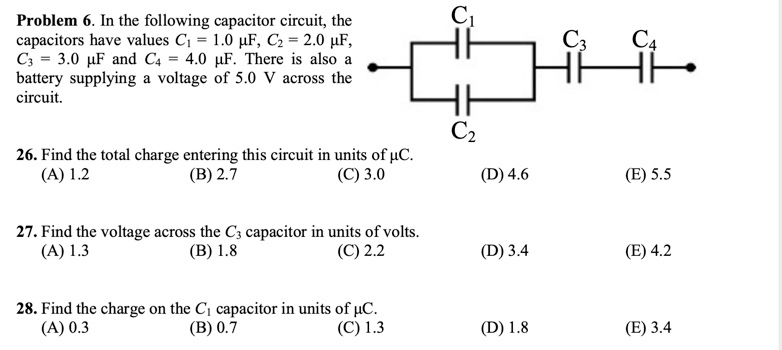Problem 6. In the following capacitor circuit, the capacitors have values C1 = 1.0 uF, C2 = 2.0 uF, C3 = 3.0 uF and C4 = 4.0 uF. There is also a battery supplying a voltage of 5.0 V across the circuit. = = 26. Find the total charge entering this circuit in units of uC. (A) 1.2 (B) 2.7 (C) 3.0 (D) 4.6 (E) 5.5 27. Find the voltage across the Cz capacitor in units of volts. (A) 1.3...

• ### A circuit is constructed with two capacitors and an inductor as shown. The values for the...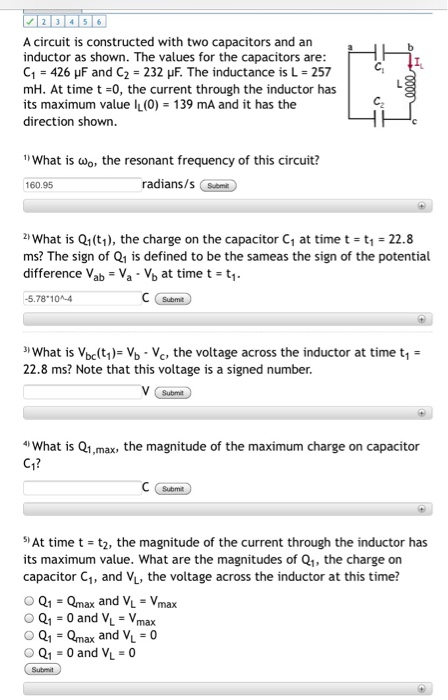A circuit is constructed with two capacitors and an inductor as shown. The values for the capacitors are: C1 = 426 uF and C2 = 232 pF. The inductance is L = 257 mH. At time t =0, the current through the inductor has its maximum value IL(0) = 139 mA and it has the direction shown. La "What is wo, the resonant frequency of this circuit? 160.95 radians/s Submit 21 What is Q1(t1), the charge on the capacitor C1...

• ### A circuit is constructed with two capacitors and an inductor as shown. The values for the...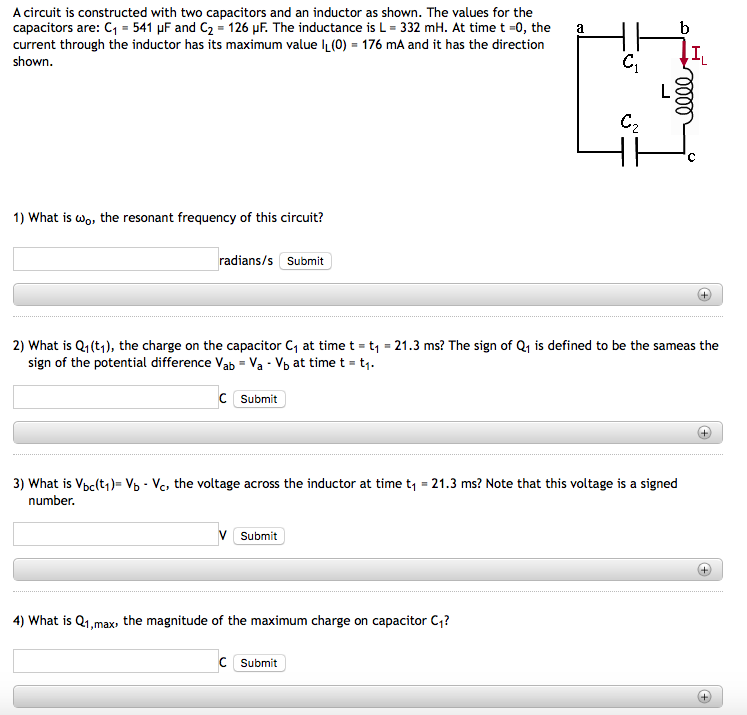A circuit is constructed with two capacitors and an inductor as shown. The values for the capacitors are: C1-541 uF and C2-126 uF. The inductance is L-332 mH. At time t -0, the current through the inductor has its maximum value IL(0)-176 mA and it has the direction shown a 1) What is ?0, the resonant frequency of this circuit? radians/s Submit 2) What is Q1(t1), the charge on the capacitor C1 at time t t 21.3 ms? The sign...

• ### The figure shows a circuit with an ideal battery and set of capacitors. The battery has...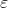The figure shows a circuit with an ideal battery and set of capacitors. The battery has an emf =12 V. The capacitors have capacitances C1 = C2 = C3 = C4 = 6 nF. (a) What is the equivalent capacitance of the entire network of capacitors? (b) What is the electrical energy stored in the capacitor with capacitance C2? We were unable to transcribe this imageProblem 6 C. The figure shows a circuit with an ideal battery and set of...

• ### Four capacitors are arranged in the circuit shown in the figure. The capacitors have the values...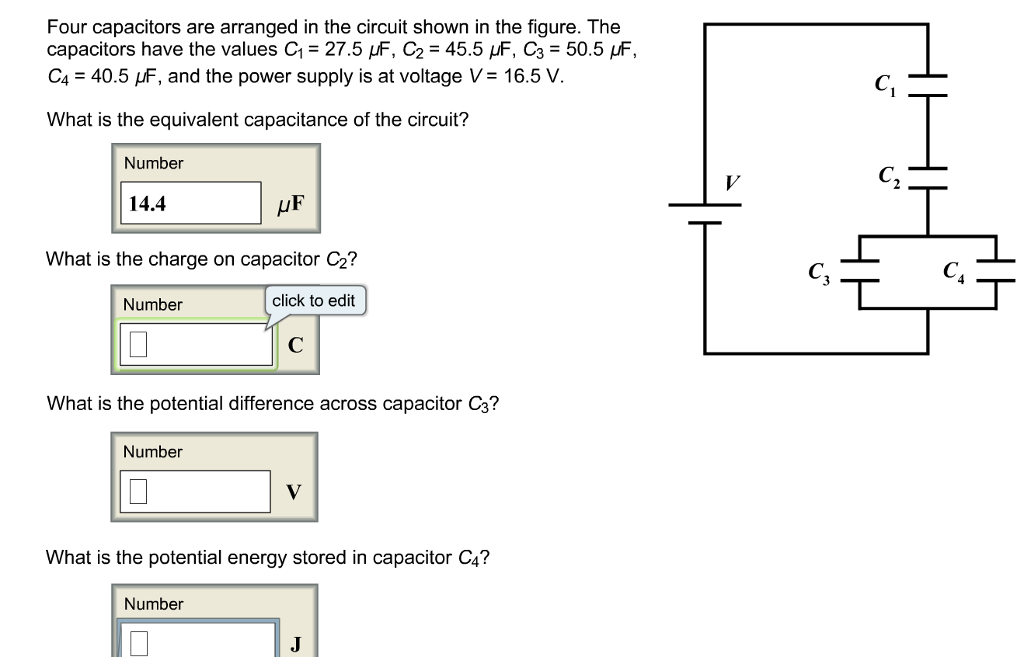Four capacitors are arranged in the circuit shown in the figure. The capacitors have the values C1 = 27.5 μF, C2 = 45.5 μF, C3 = 50.5 μF, C4 = 40.5 μF, and the power supply is at voltage V = 16.5 V. What is the equivalent capacitance of the circuit? Four capacitors are arranged in the circuit shown in the figure. The capacitors have the values C1-27.5 μF, C2 = 45.5 μF, C3 = 50.5 F, C.-40.5 and the...

• ### The circuit shown in the figure below contains three capacitors whose values are as follows: C1=1...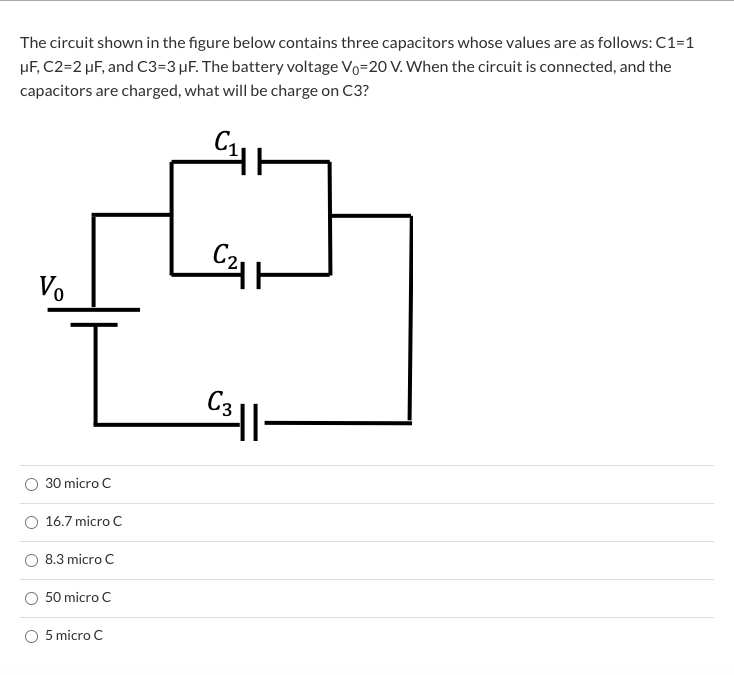The circuit shown in the figure below contains three capacitors whose values are as follows: C1=1 UF, C2=2 pF, and C3=3 uF. The battery voltage Vo=20 V. When the circuit is connected, and the capacitors are charged, what will be charge on C3? C2, V. C3 GO 30 micro C 16.7 micro C 8.3 micro C 50 micro C 5 micro C